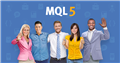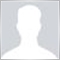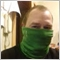# Need help with trailing stop16

`trailing stop which activates after 20 pints of profit and has to shift every 10 pips but I kicked something`

Where i do mistake ?

//+------------------------------------------------------------------+

//|                                                         od17.mq4 |
//|                        Copyright 2018, MetaQuotes Software Corp. |
//|                                             https://www.mql5.com |
//+------------------------------------------------------------------+
#property version   "1.00"
#property strict

extern int MagicNumber = 117;
extern int Slippage = 3;
extern double LotSize = 0.05;
int counter = 0;
extern int distance_between_HighLow_and_Stoploss = 0; // mozna tutaj przesunac stop loss w pipsach od jakiegos poziomu 10 = 1 pips
extern int SL_POINT = 35;
extern int TP_POINT =170;

//+------------------------------------------------------------------+
//| Expert initialization function                                   |
//+------------------------------------------------------------------+
int OnInit()
{
//---

//---
return(INIT_SUCCEEDED);
}
//+------------------------------------------------------------------+
//| Expert deinitialization function                                 |
//+------------------------------------------------------------------+
void OnDeinit(const int reason)
{
//---

}
//+------------------------------------------------------------------+
//| Expert tick function                                             |
//+------------------------------------------------------------------+
void OnTick()
{
//---
MqlTick lastTick;

if (Hour() == 13 && Minute() == 01) {
// open order
if(SymbolInfoTick(Symbol(),lastTick)) {
//Print( lastTick.bid );
if (lastTick.bid > iOpen(Symbol(), PERIOD_H1, 1)) {
} else {
sendSellOrder();
}
}

}
if (Hour() == 23 && Minute() == 57) {
// close order
manuallyCloseOrder();
}

//Print( iOpen(Symbol(), PERIOD_D1, 1) );
//Print( iLow(Symbol(), PERIOD_D1, 1) );
//Print( iHigh(Symbol(), PERIOD_D1, 1) );

}
//+------------------------------------------------------------------+
//| ChartEvent function                                              |
//+------------------------------------------------------------------+
void OnChartEvent(const int id, const long &lparam, const double &dparam, const string &sparam) {}
//+------------------------------------------------------------------+

if (OrdersTotal() > 0) return;
double minstoplevel_SL = MarketInfo(Symbol(),MODE_STOPLEVEL)+SL_POINT*10;
double minstoplevel_TP = MarketInfo(Symbol(),MODE_STOPLEVEL)+TP_POINT*10;
double stoploss = NormalizeDouble(Bid-minstoplevel_SL*Point,Digits);
double takeprofit = NormalizeDouble(Bid+minstoplevel_TP*Point,Digits);
counter++;
}

void sendSellOrder() {
if (OrdersTotal() > 0) return;
double minstoplevel_SL = MarketInfo(Symbol(),MODE_STOPLEVEL)+SL_POINT*10;
double minstoplevel_TP = MarketInfo(Symbol(),MODE_STOPLEVEL)+TP_POINT*10;
OrderSend(Symbol(), OP_SELL, LotSize, Bid, Slippage, stoploss, takeprofit,"Sell[" + counter +"]", MagicNumber, 0, clrRed);
counter++;
}

void manuallyCloseOrder() {
for(int i=OrdersTotal(); i>=0; i--) {
if(OrderSelect(i, SELECT_BY_POS)==true) {
if (OrderType() == 0) {
OrderClose(OrderTicket(), OrderLots(), MarketInfo(OrderSymbol(), MODE_BID), 0, clrNONE);
} else if (OrderType() == 1) {
OrderClose(OrderTicket(), OrderLots(), MarketInfo(OrderSymbol(), MODE_ASK), 0, clrNONE);
}
}
}
}
int startTS = 20;
int TS = 10;
int step = 20;

void trailingStop() {
for(int i=OrdersTotal(); i>=0; i--) {
if(OrderSelect(i, SELECT_BY_POS)==true) {
if (OrderType() == 0) {
double n = NormalizeDouble((Ask - OrderOpenPrice()) * getSymbolScale(), 2);
//Print( n );
double m = NormalizeDouble((Ask - OrderStopLoss()) * getSymbolScale(), 2);
//Print( " m " + m );
if (NormalizeDouble(((Bid - OrderOpenPrice()) * getSymbolScale()), 2) > startTS) {
if (m > step) {
double new_sl = NormalizeDouble(OrderStopLoss()+(MarketInfo(Symbol(),MODE_STOPLEVEL)+TS*10)*Point,Digits);
OrderModify(OrderTicket(), OrderOpenPrice(), new_sl, OrderTakeProfit(), 0, clrNONE);
}
}
} else if (OrderType() == 1) {
double n = NormalizeDouble((OrderOpenPrice() - Ask) * getSymbolScale(), 2);
//Print( n );
double m = NormalizeDouble((OrderStopLoss() - Ask) * getSymbolScale(), 2);
//Print( " m " + m );
if (NormalizeDouble(((OrderOpenPrice() - Ask) * getSymbolScale()), 2) > startTS) {
if (m > step) {
double new_sl = NormalizeDouble(OrderStopLoss()-(MarketInfo(Symbol(),MODE_STOPLEVEL)+TS*10)*Point,Digits);
OrderModify(OrderTicket(), OrderOpenPrice(), new_sl, OrderTakeProfit(), 0, clrNONE);
}
}
}
}
}
}

double getSymbolScale() {
double res = 0;
if (Point == 0.00001) { // EURUSD, GBPUSD etc
res = 10000; // ok
} else if (Point == 0.001) { // USDJPPY etc
res = 1000; //
} else if (Point == 0.01) { // DAX
res = 100; //
}
return res;
}Discover new MetaTrader 5 opportunities with MQL5 community and services
• www.mql5.com21

Move your trailing stop function into OnTick()35

1. Do you understand that you will receive many (not one) ticks on 13:01? and can get into sendBuyOrder not once?

2. you have trailingStop() routine. But where do you call for it? How does expert get into there?Moderator
20419

Do not double post.

I have deleted your other topic.16

Yaroslav Belousov:
1. Do you understand that you will receive many (not one) ticks on 13:01? and can get into sendBuyOrder not once?

2. you have trailingStop() routine. But where do you call for it? How does expert get into there?

1 no i wil not cous of if (OrdersTotal() > 0) return; - OrdersTotal() so if I have an open position it will return 1 and will not open another one. I moved the trailing stop to the void OnTick () function and it works. Now lock like this:

//+------------------------------------------------------------------+

//|                                                         od17.mq4 |
//|                        Copyright 2018, MetaQuotes Software Corp. |
//|                                             https://www.mql5.com |
//+------------------------------------------------------------------+
#property version   "1.00"
#property strict

extern int MagicNumber = 117;
extern int Slippage = 3;
extern double LotSize = 0.05;
int counter = 0;
extern int distance_between_HighLow_and_Stoploss = 0; // mozna tutaj przesunac stop loss w pipsach od jakiegos poziomu 10 = 1 pips
extern int SL_POINT = 35;
extern int TP_POINT =170;

//+------------------------------------------------------------------+
//| Expert initialization function                                   |
//+------------------------------------------------------------------+
int OnInit()
{
//---

//---
return(INIT_SUCCEEDED);
}
//+------------------------------------------------------------------+
//| Expert deinitialization function                                 |
//+------------------------------------------------------------------+
void OnDeinit(const int reason)
{
//---

}
//+------------------------------------------------------------------+
//| Expert tick function                                             |
//+------------------------------------------------------------------+
void OnTick()
{
//---
MqlTick lastTick;

if (Hour() == 13 && Minute() == 01) {
// open order
if(SymbolInfoTick(Symbol(),lastTick)) {
//Print( lastTick.bid );
if (lastTick.bid > iOpen(Symbol(), PERIOD_H1, 1)) {
} else {
sendSellOrder();
}
}

}
if (Hour() == 23 && Minute() == 57) {
// close order
manuallyCloseOrder();
}

int startTS = _startTS;
int TS = _TS;
int step = _step;

for(int i=OrdersTotal(); i>=0; i--) {
if(OrderSelect(i, SELECT_BY_POS)==true) {
if (OrderType() == 0) {
double n = NormalizeDouble((Ask - OrderOpenPrice()) * getSymbolScale(), 2);
//Print( n );
double m = NormalizeDouble((Ask - OrderStopLoss()) * getSymbolScale(), 2);
//Print( " m " + m );
if (NormalizeDouble(((Bid - OrderOpenPrice()) * getSymbolScale()), 2) > startTS) {
if (m > step) {
double new_sl = NormalizeDouble(OrderStopLoss()+(MarketInfo(Symbol(),MODE_STOPLEVEL)+TS*10)*Point,Digits);
OrderModify(OrderTicket(), OrderOpenPrice(), new_sl, OrderTakeProfit(), 0, clrNONE);
}
}
} else if (OrderType() == 1) {
double n = NormalizeDouble((OrderOpenPrice() - Ask) * getSymbolScale(), 2);
//Print( n );
double m = NormalizeDouble((OrderStopLoss() - Ask) * getSymbolScale(), 2);
//Print( " m " + m );
if (NormalizeDouble(((OrderOpenPrice() - Ask) * getSymbolScale()), 2) > startTS) {
if (m > step) {
double new_sl = NormalizeDouble(OrderStopLoss()-(MarketInfo(Symbol(),MODE_STOPLEVEL)+TS*10)*Point,Digits);
OrderModify(OrderTicket(), OrderOpenPrice(), new_sl, OrderTakeProfit(), 0, clrNONE);
}
}
}
}
}

//Print( iOpen(Symbol(), PERIOD_D1, 1) );
//Print( iLow(Symbol(), PERIOD_D1, 1) );
//Print( iHigh(Symbol(), PERIOD_D1, 1) );

}
//+------------------------------------------------------------------+
//| ChartEvent function                                              |
//+------------------------------------------------------------------+
void OnChartEvent(const int id, const long &lparam, const double &dparam, const string &sparam) {}
//+------------------------------------------------------------------+

{
if (OrdersTotal() > 0) return;
double minstoplevel_SL = MarketInfo(Symbol(),MODE_STOPLEVEL)+SL_POINT*10;
double minstoplevel_TP = MarketInfo(Symbol(),MODE_STOPLEVEL)+TP_POINT*10;
double stoploss = NormalizeDouble(Bid-minstoplevel_SL*Point,Digits);
double takeprofit = NormalizeDouble(Bid+minstoplevel_TP*Point,Digits);
counter++;
}

void sendSellOrder()
{
if (OrdersTotal() > 0) return;
double minstoplevel_SL = MarketInfo(Symbol(),MODE_STOPLEVEL)+SL_POINT*10;
double minstoplevel_TP = MarketInfo(Symbol(),MODE_STOPLEVEL)+TP_POINT*10;
OrderSend(Symbol(), OP_SELL, LotSize, Bid, Slippage, stoploss, takeprofit,"Sell[" + counter +"]", MagicNumber, 0, clrRed);
counter++;
}

void manuallyCloseOrder() {
for(int i=OrdersTotal(); i>=0; i--) {
if(OrderSelect(i, SELECT_BY_POS)==true) {
if (OrderType() == 0) {
OrderClose(OrderTicket(), OrderLots(), MarketInfo(OrderSymbol(), MODE_BID), 0, clrNONE);
} else if (OrderType() == 1) {
OrderClose(OrderTicket(), OrderLots(), MarketInfo(OrderSymbol(), MODE_ASK), 0, clrNONE);
}
}
}
}
double getSymbolScale() {
double res = 0;
if (Point == 0.00001) { // EURUSD, GBPUSD etc
res = 10000; // ok
} else if (Point == 0.001) { // USDJPPY etc
res = 1000; //
} else if (Point == 0.01) { // DAX
res = 100; //
}
return res;

}

`And now I have a question for you how to make a sl = 50% of the last candle from h1`Discover new MetaTrader 5 opportunities with MQL5 community and services
• www.mql5.com
Ask questions on technical analysis, discuss trading systems and improve your MQL5 programming skills to develop your own trading strategies. Communicate and share your experience with traders from anywhere in the world, answer questions and help beginners — MQL5.community is developing along with you. Multiple accounts EA's and Signals Hi...